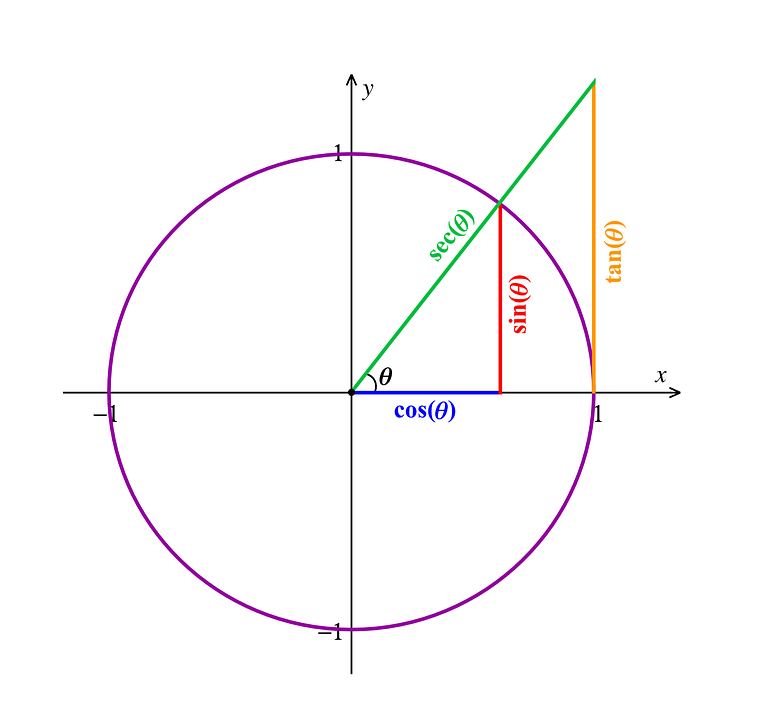# What Is Trigonometry? Formula, Examples, Applications, TablesAcemyhomework BlogAlgebra, geometry, probability, trigonometry, arithmetic, and other fields of mathematics are among the most significant. On the other hand, trigonometry is a subset of geometry concerned with the properties of one of the shapes in geometry known as a "triangle." Although trigonometry and geometry appear to be linked, they are not the same. Let us look at what trigonometry is in detail through this post.

### What Is Trigonometry in Simple Words?

One of the most significant branches of mathematics is trigonometry. The terms 'Trigonon' and 'Metron,' which imply triangle and measure respectively, are combined to form trigonometry. It studies the relationship between a right-angled triangle's sides and angles. It thus facilitates the use of formulas and identities based on this relationship to find the measure of unknown sides of a right-angled triangle.

### What Is the Difference Between Geometry and Trigonometry?

The study of varied sizes, shapes, and qualities of vacant spaces of a certain number of dimensions, such as 2D or 3D, is known as geometry. At the same time, trigonometry is extremely important in modern mathematics. Trigonometry is primarily concerned with studying triangles, lengths, and angles. It does deal with oscillations and waves.

In trigonometry, we primarily study the relationships between a right-angle triangle's side lengths and angles. There are six trigonometric relations. Sine, Cosine, and Tangent are three basic ones, which are put together with Secant, Cosecant, and Cotangent.

#### What Is Trigonometry Used For

Trigonometry has three essential functions: sine, cosine, and tangent. Other vital trigonometric functions can be derived using these three fundamental ratios or functions: cotangent, secant, and cosecant. These uses form the foundation for all of the essential topics in trigonometry. As a result, to comprehend trigonometry, we must first study these functions and their formulas.

Three sides of a right-angled triangle are:

• The opposite side to the angle is known as perpendicular.
• The angle's adjacent side is the base.
• The hypotenuse is opposite the right angle.

Trigonometry has been used in various fields throughout history, including

• Architects, celestial mechanics, and surveying all use trigonometry.
• Its applications are oceanography, seismology, meteorology, physical sciences, astronomy, acoustics, navigation, electronics, and many more.
• Spherical trigonometry is used to locate solar, lunar, and stellar locations, as well as to estimate the distance between lengthy rivers, measure the height of a mountain, and so on.
• Trigonometry is used in criminology to estimate the cause of collision and trajectory of bullets.
• Marine biologists also use trigonometry to establish the depth of sunlight that triggers algae to photosynthesis.
• Trigonometry in Aviation is used to calculate the direction a pilot can turn to avoid a collision or strong wind turbulence.
• Trigonometry in navigation is applied when the sailor uses angles to navigate to the correct destinations.

### Trigonometry in the real World

Most real-life examples use trigonometry.

A young boy stands at the side of a tree. “How tall is the tree?" The boy wonders, the tree's height is easily determined without measuring it. A right-angled triangle is what we have here.

#### What Is Sine in Trigonometry?

The ratio distance of one side of the triangle opposite the angle to the length of the triangle's hypotenuse is the sine of one of the angles of a right triangle (commonly abbreviated "sin").

Sin θ = Perpendicular/Hypotenuse

#### What Is Tan in Trigonometry?

The trigonometric ratio between the adjacent and opposite sides of a right-angled triangle comprising that angle is the tangent of an angle.

Tan θ = Perpendicular/Base

### Important Trigonometric Angles

Trigonometric angles are in a right-angled triangle and can represent different trigonometric functions. Some angles in trigonometry include 0º, 30º, 45º, 60º, 90º. The values of these angles can be observed directly in a trigonometric table. With some other vital angles like 180º, 270º, and 360º. Trigonometry angle is expressed in terms of trigonometric ratios as,

### Trigonometric Ratios

Six fundamental ratios in trigonometry help establish the ratio of sides of a right triangle with the angle.

Sin θ = Perpendicular/Hypotenuse

Cos θ = Base/Hypotenuse

Tan θ = Perpendicular/Base.

Cot θ = 1/tan θ = Base/Perpendicular

Sec θ = 1/cos θ = Hypotenuse/Base

Cosec θ = 1/sin θ = Hypotenuse/Perpendicular

### What Is the Trigonometry Formula?

Many formulas show the relationships between trigonometric ratios and angles for various quadrants in trigonometry. The following is a list of basic trigonometry formulas:

#### 1. Trigonometry Ratio Formulas

Sin θ = Hypotenuse/Opposite side

Cot θ = 1/tan θ = Opposite Side/Adjacent

Sec θ = 1/cos θ = Adjacent Side/Hypotenuse

Cosec θ = 1/sin θ = Opposite Side/Hypotenuse

θ = sin-1 (Perpendicular/Hypotenuse)

θ = cos-1 (Base/Hypotenuse)

θ = tan-1 (Perpendicular/Base)

#### 2. Trigonometry Formulas with Pythagorean Identities

cos²θ+sin²θ = 1

1+tan2θ = sec2θ

1+cot2θ = cosec2θ

3. Cosine and cot2θ Law in Trigonometry

A/sinA = b/sinB = c/sinC

c2 = a2 + b2 – 2ab cos C

a2 = b2 + c2 – 2bc cos A

b2 = a2 + c2 – 2ac cos B

### Trigonometric Table

The trigonometric table consists of connected ratios such as sine, cosine, tangent, cosecant, secant, and cotangent. These ratios are sin, cos, tan, cosec, sec, and cot and calculate standard angle values. To learn more about these ratios, look at the trigonometry table below.

With the information provided about trigonometry, it is clear that dealing with angles involves more mathematics than meets the eye. At Acemyhomework, we pride in making school work an easy and manageable task for every student, we have professional writers who are available around the clock to ensure that you receive the best writing services.